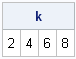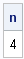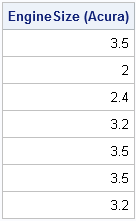A fundamental operation in data analysis is finding data that satisfy some criterion. How many people are older than 85? What are the phone numbers of the voters who are registered Democrats? These questions are examples of locating data with certain properties or characteristics.

The SAS DATA step has a variety of statements (such as the WHERE and IF statements) that enable statistical programmers to locate observations and subset data. The SAS/IML language has similar language features, but because data is often stored in SAS/IML matrices, the SAS/IML language also has a function that is not available in the DATA step: the LOC function.

### The LOC Function

If your data are in a SAS/IML matrix, x, the LOC Function enables you to find elements of x for which a given criterion is true. The LOC function returns the LOCations (indices) of the relevant elements. (In the R language, the which function implements similar functionality.) For example, the following statements define a numeric vector, x, and use the LOC function to find the indices for which the numbers are greater than 3:

```proc iml; x = {1 4 3 5 2 7 3 5}; /** which elements are > 3? **/ k = loc( x>3 ); print k;```Notice the following:

• The argument to the LOC function is an expression that resolves to a vector of 0s and 1s. (Some languages call this a logical vector.) In practice, the argument to the LOC function is almost always an expression.
• The result of the LOC function is always a row vector. The number of columns is the number of elements of x that satisfy the given criterion.
• The LOC function returns indices of x, not values of x. To obtain the values, use x[k]. (Indices and subscripts are related; for vectors, they are the same.)

### How Many Elements Satisfy the Criterion?

You can exploit the fact that the LOC function outputs a row vector. To count the number of elements that satisfy the criterion, simply use the NCOL function, as follows:

```n = ncol(k); /** how many? **/ print n;```### What If No Elements Satisfy the Criterion?

The expression ncol(idx) always tells you the number of elements that satisfy the criterion, even when no elements satisfy the criterion. The following statement asks for the elements larger than 100 and handles the possible results:

```j = loc( x>100 ); if ncol(j) > 0 then do; print "At least one element found"; /** handle this case **/ end; else do; print "No elements found"; /** handle alternate case **/ end;```

In the preceding example, x does not contain any elements that are greater than 100. Therefore the matrix j is an empty matrix, which means that j has zero rows and zero columns. It is a good programming practice to check the results of the LOC function to see if any elements satisfied the criterion. For more details, see Chapter 3 of Statistical Programming with SAS/IML Software.

### Using the LOC Function to Subset a Vector

The LOC function finds the indices of elements that satisfy some criterion. These indices can be used to subset the data. For example, the following statements read information about vehicles in the SasHelp.Cars data set. The READ statement creates a vector that contains the make of each vehicle ("Acura," "Audi," "BMW,"...) and creates a second vector that contains the engine size (in liters) for each vehicle. The LOC function is used to find the indices for the vehicles made by Acura. These indices are then used to subset the EngineSize vector in order to produce a vector, s, that contains only the engine volumes for the Acura vehicles:

```use sashelp.cars; read all var {Make EngineSize}; close sashelp.cars;   /** find observations that satisfy a criterion **/ idx = loc( Make="Acura" ); s = EngineSize[idx]; print s[label="EngineSize (Acura)"];```### LOC = Efficient SAS Programming

I have called the LOC function the most useful function that most DATA step programmers have never heard of. Despite its relative obscurity, it is essential that SAS/IML programmers master the LOC function. By using the LOC function, you can write efficient vectorized programs, rather than inefficient programs that loop over data.

ShareDistinguished Researcher in Computational Statistics

Rick Wicklin, PhD, is a distinguished researcher in computational statistics at SAS and is a principal developer of SAS/IML software. His areas of expertise include computational statistics, simulation, statistical graphics, and modern methods in statistical data analysis. Rick is author of the books Statistical Programming with SAS/IML Software and Simulating Data with SAS.

1.Thanks for an excellent blog! A simple question related to the loc.

Say you have a marginal probability matrix M = {prob a b c} which represent the probability of "a" conditional on "b" and "c" (all categorical variables). You would like to draw the sub-matrix of conditional prob of a when "b"=i and "c"=j. This is something you need in a Gibbs sampling sort-of-exercise. Is there an efficient way that I have overlooked?

Best
/J

•Post your question with an example matrix to the SAS/IML Support Community.

2.Hi Rick,

Your website is very helpful! I tried to use "LOC" but it doesn't give me the answer. Could you take a look? Maybe the vector is too long??

%let cut = 0.84;
proc iml ;
the = do(-4, 4, 0.1);
jcut=loc(the = &cut.);
print jcut;

Thank you so much for your help!

Best,
Sunhee

•The value 0.84 is not contained in the vector, but even if you change &cut to be 0.8 you are not likely to find 0.8 because of the numerical representation of values in finite precision arithmetic. For example, run this code:
diff = 0.8 - the; print diff;

Your example is basically the same as the first example in this article about why you should avoid testing for equality in finite precision computations. For comparisons like this, you can round the computed results before you test for equality, as follows:
the = round(the, 1e-8);